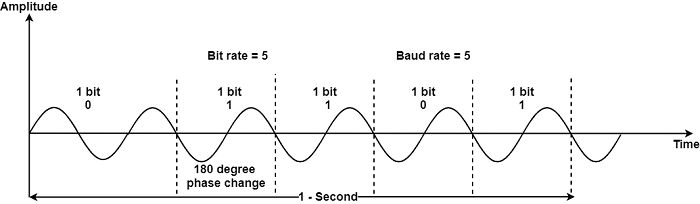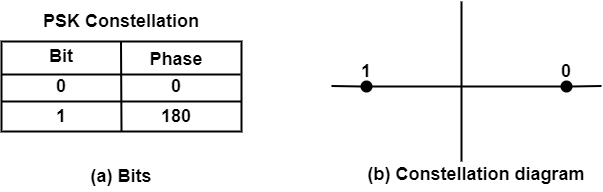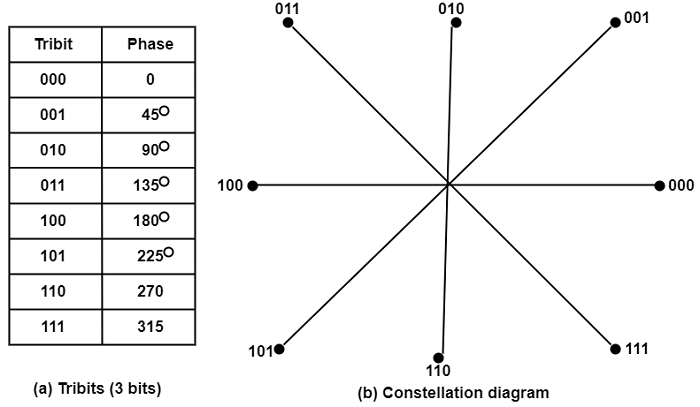# What is Phase Shift Keying (PSK) in Computer Network?

Computer NetworkNetworkOperating System

#### Artificial Neural Network and Machine Learning using MATLAB

54 Lectures 4 hours

In phase-shift keying (PSK), the carrier phase is different to describe binary 1 or 0. Both peak amplitude and frequency remain constant as the process modifies. For example, if we begin with 0 degrees to define binary 0, we can adjust the procedure to 180 degrees to transmit binary 1. During each bit duration, the signal phase is fixed, and its cost is based on the bit (0 or 1).

The diagram shows a conceptual view of PSK −The following technique is known as 2-PSK, or binary PSK because two multiple procedures (0 and 180 degrees) are used. Figures make this point clearer by displaying the relationship of the process to a bit value. A second diagram referred to as constellation or phase-state diagram, shows a similar relationship by representing only the phases.PSK is not affected by the noise degradation that change ASK or the bandwidth drawback of FSK. This defines the find accurately by the receiver. Hence, rather than using only two alterations of a signal, each describing one bit, we can use four variations and let each procedure transfer define two bits.

The constellation figure for the signal is given in the diagram. The 4-PSK phase of 0 degrees now describe 00, 90 degrees describe 01, 180 degrees describe 10, and 270 degrees describe 11. This method is called 4-PSK or Q-PSK. The pair of bits defined by each phase is known as dibit. We can transmit data two times as quick using 4-PSK as we can use 2-PSK. Further, this concept can be to 8-PSK.

In place of now, the signal can be varied by shifts of With eight different phases, each transition can represent 3 bits at a time. It can be observed that the relationship of the number of bits per shift to the number of phases is a power of 2. When we have 8 possible phases, we can transmit 3 bits at a time, i.e.,

The diagram displays the relationships between the phase shifts and the tribits. Each one represents 8-PSK in 3 times as efficient as 2-PSK.### Bandwidth for Phase Shift Keying (PSK)

The minimum bandwidth needed for PSK transmission is similar to that needed for ASK transmission. The maximum bit rate in PSK transmission is probably much higher than ASK. Therefore, while the maximum band rates of ASK and PSK are similar for a provided bandwidth, PSK bit rates using the equal bandwidth can be 2 or more times higher.